# Solar Wind Data

### Solar Wind Data

Solar Wind data are courtesy of OMNI (https://omniweb.sci.gsfc.nasa.gov/ow_min.html), Dr. Natalia Papitashvili, Mail Code 672, NASA/Goddard Space Flight Center, Greenbelt, MD 20771. SuperMAG use the high-time resolution dataset that are “1-min-averaged, field/plasma data sets shifted to the Earth's bow shock nose (BSN). This "High Resolution OMNI" (HRO) data set involves an interspersal of BSN-shifted ACE, Wind, IMP 8 and Geotail data. The following material describes the content and building of this HRO data set and related data sets.”

If used in publications and/or presentations please fully acknowledge OMNI: https://omniweb.sci.gsfc.nasa.gov/html/citing.html

Data gaps are indicated by 999999.

Clock angle: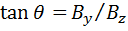where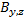is the interplanetary magnetic field y and z component (GSM coordinates).  Units are degrees.

The Epsilon Parameter: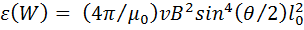where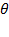is the clock angle,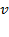is the solar wind speed, and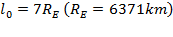.  Units are GW.

The Newell Coupling Function: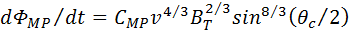whereis the clock angle,is the solar wind speed, and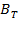is the interplanetary field magnitude. Units are Wb/s. The original function has been converted to Wb/s by multiplying by CMP = 100 to convert the units to Wb/s (based on the observational estimate made by Cai and Clauer ).

Dynamic Pressure: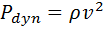where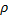is the mass density.  We assume charge neutrality and that the ions and electrons have identical flow velocities.  Further assuming that the ions in the solar wind consist of 96% H+ and 4% He++ ions.  Hence for 1000 electrons there are 923 protons and 77 He ions.  Units are nPa.

### References

Akasofu, S.-I. (1981) Energy coupling between the solar wind and the magneto- sphere, Space Sci. Rev., 28, 121.

Cai, X., and Clauer C. R. (2013), Magnetospheric sawtooth events during the solar cycle 23, J. Geophys. Res.: Space Physics, 118, 6378-6388, doi:10.1002/2013JA018819.

Newell, P. T., T. Sotirelis, K. Liou, C.-I. Meng, and F. J. Rich (2007), A nearly universal solar wind-magnetosphere coupling function inferred from 10 magnetospheric state variables, J. Geophys. Res., 112, A01206, doi:10.1029/2006JA012015.

Perreault, P., and S.-I. Akasofu (1978), A study of geomagnetic storms, Geophys. J. R. Astron. Soc., 54, 547–573.

Weimer, D.R., D.M. Ober, N.C. Maynard, M.R. Collier, D.J. McComas, N.F. Ness, C.W. Smith, and J. Watermann (2003), Predicting interplanetary magnetic field (IMF) propagation delay times using the minimum variance technique, J. Geophys. Res., 108, doi:10.1029/2002JA009405.

Weimer, D. R. (2004), Correction to ''Predicting interplanetary magnetic field (IMF) propagation delay times using the minimum variance technique’', J. Geophys. Res., 109, A12104, doi:10.1029/2004JA010691.